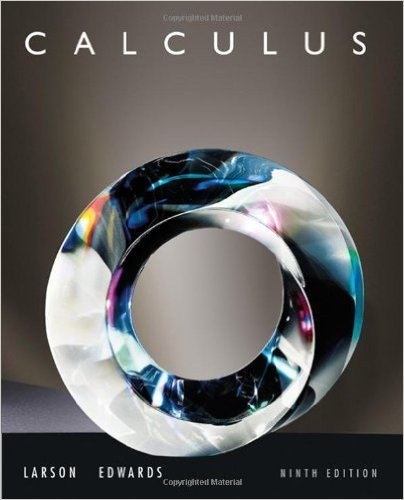×
×

# Solutions for Chapter 3.6: A Summary of Curve Sketching## Full solutions for Calculus | 9th Edition

ISBN: 9780547167022Solutions for Chapter 3.6: A Summary of Curve Sketching

Solutions for Chapter 3.6
4 5 0 264 Reviews
20
0
##### ISBN: 9780547167022

Calculus was written by and is associated to the ISBN: 9780547167022. This textbook survival guide was created for the textbook: Calculus , edition: 9. Chapter 3.6: A Summary of Curve Sketching includes 79 full step-by-step solutions. Since 79 problems in chapter 3.6: A Summary of Curve Sketching have been answered, more than 63178 students have viewed full step-by-step solutions from this chapter. This expansive textbook survival guide covers the following chapters and their solutions.

Key Calculus Terms and definitions covered in this textbook
• Angle of depression

The acute angle formed by the line of sight (downward) and the horizontal

• Coordinate(s) of a point

The number associated with a point on a number line, or the ordered pair associated with a point in the Cartesian coordinate plane, or the ordered triple associated with a point in the Cartesian three-dimensional space

• Ellipse

The set of all points in the plane such that the sum of the distances from a pair of fixed points (the foci) is a constant

• Fitting a line or curve to data

Finding a line or curve that comes close to passing through all the points in a scatter plot.

• Graph of a function ƒ

The set of all points in the coordinate plane corresponding to the pairs (x, ƒ(x)) for x in the domain of ƒ.

• Graphical model

A visible representation of a numerical or algebraic model.

• Hyperboloid of revolution

A surface generated by rotating a hyperbola about its transverse axis, p. 607.

• Integrable over [a, b] Lba

ƒ1x2 dx exists.

• Monomial function

A polynomial with exactly one term.

• Open interval

An interval that does not include its endpoints.

• Quotient of functions

a ƒ g b(x) = ƒ(x) g(x) , g(x) ? 0

• Range (in statistics)

The difference between the greatest and least values in a data set.

• Reflection through the origin

x, y and (-x,-y) are reflections of each other through the origin.

• Sample space

Set of all possible outcomes of an experiment.

• Scatter plot

A plot of all the ordered pairs of a two-variable data set on a coordinate plane.

• Sequence of partial sums

The sequence {Sn} , where Sn is the nth partial sum of the series, that is, the sum of the first n terms of the series.

• Trigonometric form of a complex number

r(cos ? + i sin ?)

• Variable (in statistics)

A characteristic of individuals that is being identified or measured.

• Xmin

The x-value of the left side of the viewing window,.

• yz-plane

The points (0, y, z) in Cartesian space.

×